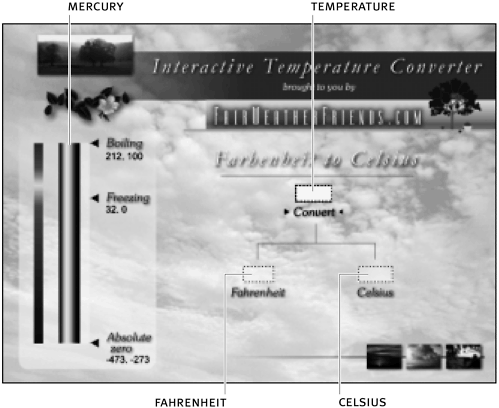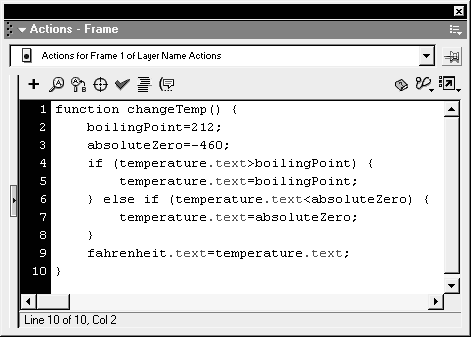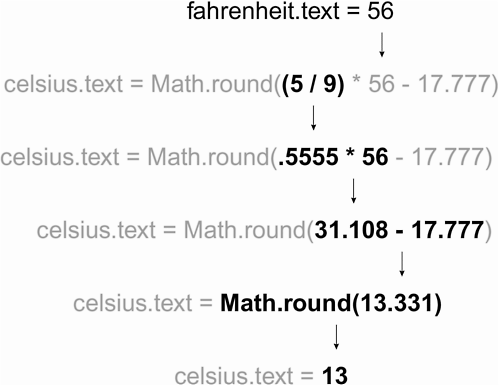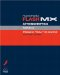# MANIPULATING NUMERICAL DATA USING MATH

 Earlier in this lesson, we introduced you to the numeric operators, which allow you to perform simple arithmetic in your expressions. Flash's Math object allows you to access a variety of useful methods that allow you to further manipulate numbers. We'll introduce you to a few of the most commonly used methods of the Math object here; you'll use many of the other methods in other lessons in this book. Common methods of the Math object include the following: Math.abs() The absolute-value method is used to return the scalar (positive) value of a number. Example: distance = Math.abs(here there); If subtracting the value of there from here results in a negative value (for example, 375), the Math.abs method will convert it to a positive value (for example, 375), ensuring a positive result. Math.round() The round method accepts a number as a parameter and returns an integer. If the digit in the tenth placeholder of the number is 5 or greater, the number is rounded to the next highest integer; if it's less than 5, it's rounded to the next lowest integer. Example: cupsOfCoffee = Math.round(3.7); Since 7 is greater than or equal to 5, this number is rounded up to the next highest integer, or 4. Math.floor() This method works like Math.round() except that it always rounds down to the next lowest integer. Math.ceil() This method works like Math.round() except that it always rounds up to the next highest integer. Math.sqrt() The square-root method accepts a positive number as an argument and returns the square root of that number. Example : answer = Math.sqrt(9); answer is assigned a value of 3. In this exercise, using operators, expressions and Math object methods, you will write a simple algorithm that will convert Fahrenheit temperatures to Celsius. You will also program a thermometer to display the correct mercury level. Open tempConverter1.fla in the Lesson08/Assets folder. All of the symbols and text fields have been created and are on the stage so that we can focus on ActionScript. The main timeline contains four layers: Actions, Thermometer, Temperature Input, and Background. The Background layer contains the main graphics. The Temperature Input layer contains an input-text field with an instance name of temperature. This is where the temperature to be converted will be input. This layer contains also two additional text fields fahrenheit and celsius which will be used to display those values. Also on this layer is a button containing the text "Convert." The Thermometer layer contains a movie clip instance named mercury that will be scaled vertically to indicate the proper temperature. We'll use the Actions layer in a moment.With the Actions panel open, select Frame 1 of the Actions layer and add the following script: ` function changeTemp () { } ` The above script represents the beginning of a function definition. Ultimately, this function will be executed when the Convert button is pressed, and it will do the following: Convert a Fahrenheit value to Celsius. Make sure that the Fahrenheit value entered to convert is within the range on the thermometer. Scale the mercury on the thermometer to the correct height. At the beginning of the function definition you started in the preceding step, create the following variables: ` boilingPoint = 212; absoluteZero = -460; ` Our thermometer covers a large temperature range from absolute zero (approximately -460 degrees Fahrenheit) to the boiling point of water (212 degrees Fahrenheit), temperatures that will be treated as the highest and lowest acceptable input temperatures. To ensure that the input temperature is within acceptable limits, add the following script just below absoluteZero = -460 : ` if (temperature.text > boilingPoint) { temperature.text = boilingPoint; } else if (temperature.text < absoluteZero) { temperature.text = absoluteZero; } fahrenheit.text=temperature.text; `This part of the function is an if /else if statement that acts as a data filter. It says that if the value the user inputs (temperature.text ) is greater than the value of boilingPoint (which is 212), the user-input value will be automatically set to the value of boilingPoint . Otherwise, if the user inputs a value less than absoluteZero (-460), that value will be automatically set to the value of absoluteZero . After the filter, the value of temperature.text is set to display in the fahrenheit text field instance. Filter statements such as the above are used frequently in programming since it's rare for a function to accept all possible input extremes. Just below the statement we added above, add the following expression, which is used to convert Fahrenheit to Celsius: ` celsius.text = Math.round((5 / 9) * fahrenheit.text 17.777); ` The expression to the right of the equals sign converts a Fahrenheit value to Celsius. This expression sets the value of celsius.text , which is the name of the corresponding text field on the stage. Thus, this line is used to display the converted value in that text field. Notice the use of parentheses in the expression. As Flash executes this line of code, it will evaluate the first part of the expression that it can find that is fully enclosed in parentheses (in this case, 5 / 9 ). Flash then performs the multiplication, then the subtraction. The Math.round() method is not invoked until its entire argument is evaluated and replaced with a numeric result. This method will then round the value to the next integer up or down depending on the value in the tenth place of the argument.Add the following lines to the end of the current script: ` scalePercent = (Math.abs(absoluteZero Fahrenheit.text) / Math.abs(absoluteZero boilingPoint)) * 100; mercury._yScale=scalePercent; ` These two lines of code first create a variable to store the percent used to scale the mercury movie clip instance, then use that value to scale it. The expression used to set the value of scalePercent is based on the ratio of the difference between absolute zero and the temperature submitted when compared against the full temperature range. To help you understand this, let's assume that the user has entered a value of 56 in the fahrenheit text field. We know that absoluteZero equals -460 and that boilingPoint equals 212. Thus, the expression is evaluated as follows: ` (Math.abs(-460 56) / (Math.abs(-460 212)) * 100; ` or ` (Math.abs(-516) / (Math.abs(-672)) * 100; ` or ` ((516) / (672)) * 100; ` or ` (.767) * 100 ` or ` 76.7 ` The absolute-value method of the Math object is used here to ensure that the expression evaluates to a positive percentage value. (A negative percentage value wouldn't make sense in the context of this script.) The second line of the above script scales the mercury movie clip instance vertically using the value of scalePercent . Thus, the instance is scaled to 76.7 percent of its original value, which works well because the mercury movie clip was built in Flash to be the maximum height when at normal size (100 percent).With the Actions panel open, select the Convert button and add the following script: ` on (release, keyPress "") { changeTemp(); } ` When the button is released or the Enter key pressed, the changeTemp() function you just built will be called. Choose Control > Test Movie. Enter a temperature value and press the button. When the button is pressed, you'll see the mercury movie clip scale appropriately. The celsius and fahrenheit fields on the screen will also get populated with data. Try entering a temperature that falls outside the acceptable range, and you'll see that the filter if /else if statement catches and replaces it with either the upper or lower boundary. Close the test movie and save your work as tempConverter2.fla. You have now used the Math object in an expression. With it, you converted Fahrenheit to Celsius and scaled a movie clip based on a percent calculated.Macromedia Flash MX ActionScripting: Advanced Training from the Source
ISBN: 0201770229
EAN: 2147483647
Year: 2002
Pages: 161

flylib.com © 2008-2017.
If you may any questions please contact us: flylib@qtcs.net